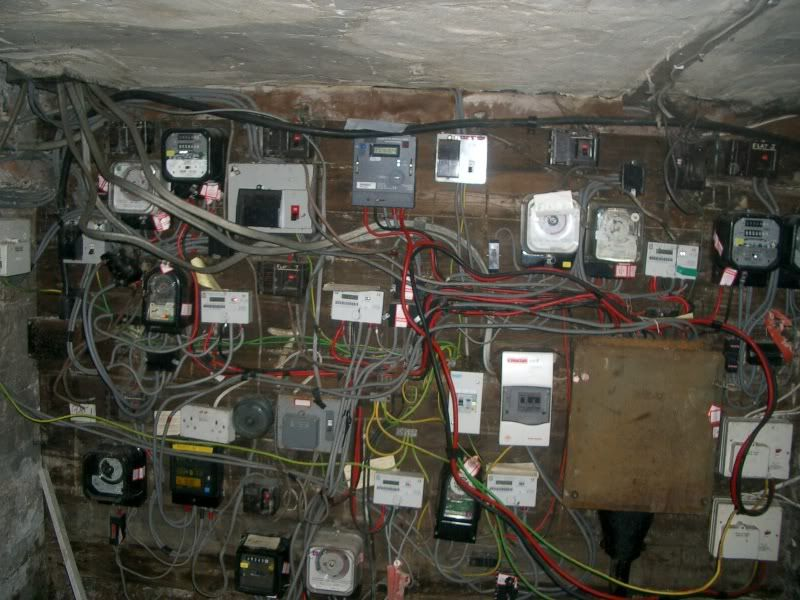# Practical inspection and testing on rig

#### Spark123

^are you sure on the P-P resistance?
What are the results you are talking of?
You need to take the end to end resistances of each conductor i.e. r1, r2, rn. Then you connect one end of r1 to the other end of rn, the other end of rn to the other end of r1. Now do the R1+Rn at each socket, should be relatively the same but for spurs.
Now interconnect r1+r2, they should all be similar (unless parallel paths exist i.e. in metal conduit etc), and at spurs. The largest figure is recorded as R1+R2.
Where is the 9.2 ohm result from - might be a bit of a problem!

#### rockenroll

I ve got, end to end for R1,Rn,R2 which is P-P is R1 loop,N-N is Rn loop, CPC-CPC is R2 loop,after, R1-N2, R2-N1 measure at sockets outlets-record values, after R1-CPC2, R2-CPC1 measure at sockets outlets-record values, I think the highest values were because of resistance what they have mounted on PVC trunkingWhat should be write on schedule of test result if you pick it up high values, how was for this ring finalCheers

#### rockenroll

The results were for 5 socket outlets between R1-Rn and R1-R2, where the values between R1-Rn and R1-R2 was approximatly almost the same...

Thank u

#### ban-all-sheds

"Welcome to the 2391 practical exam.#### Spark123

I ve got, end to end for R1,Rn,R2 which is P-P is R1 loop,N-N is Rn loop, CPC-CPC is R2 loop,after, R1-N2, R2-N1 measure at sockets outlets-record values, after R1-CPC2, R2-CPC1 measure at sockets outlets-record values, I think the highest values were because of resistance what they have mounted on PVC trunkingWhat should be write on schedule of test result if you pick it up high values, how was for this ring finalCheers

Use the smallcase r1, r2, rn for the end to end resistances.
(r1+r2)/4 = the approximate resistance you should measure at each socket around the ring (except spurs). If all your end to end resistances are 0.07 ohms then you should be measuring (0.07+0.07)/4 = 0.035 at each socket on the ring, and your ring is less than 10m long.